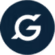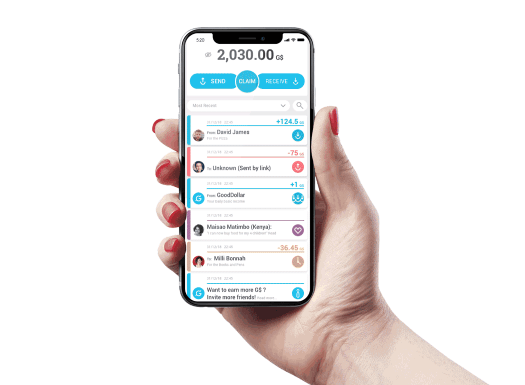How Many GoodDollar Coins Do I Receive Every Day?

# How Many GoodDollar Coins Do I Receive Every Day?gooddollar
September 7, 2020How Many GoodDollar Coins Will You Receive?

### The Short Answer To This Much-Asked Question About How Many GoodDollar Coins A Claimer Gets On A Daily Basis Is: It Changes …

One of the most common questions users have is around how many GoodDollar Coins (G\$) they will receive every day.

The answer is that every day the amount of G\$ received will vary. This is because the number of G\$ distributed to Claimers every day is determined by two parameters: the number of G\$ minted on that day, and the number of “active claimers” in the GoodDollar system.

At a high level, every day there are a number of G\$ minted that are to be distributed as basic income. This basic income pool of G\$ is divided equally between all active GoodDollar Claimers. Every day the number of GoodDollars minted may vary slightly, and every day the number of active users varies slightly – this is why every day the amount of G\$ you receive is slightly different.

Want to get into the technical details? Let’s dig in.

#### GoodDollar Minting

G\$ is a reserve-backed token, and the GoodReserve – the monetary reserve that backs GoodDollar – mints new G\$ coins in two ways (formulas can be found at the end of this blog post):

1. Interest deposited in the reserve

Every day there is interest that is generated and moved into the GoodReserve. Every time that interest is deposited, a certain number of G\$ coins are minted to keep the price stable at the moment of minting. This specific action does not change the price of G\$. (See Equation 5 below.)

1. Declining reserve ratio / expansion rate

The expansion rate is the rate of minting relative to the amount of value locked in the GoodReserve. The rate of minting is also designed to keep the price stable at the moment of minting. The initial expansion rate for the GoodDollar system was set to 20%. That means that by the end of the first year, the reserves ratio will be 80%. (See Equation 4 below.)

More on the monetary tools to manage G\$ are found in the GoodDollar White Paper.

Let’s go through a numerical example to see how it works – please bear in mind that all numbers and values presented here are for illustration purposes only.

Assumptions

• Price of 1G\$ = US\$1
• Initial Supply of G\$ (S) = 180,000
• Initial Reserve (R) = US\$90,000
• Initial Reserve Ratio (Rr) = 0.5

Mint from Interest Deposited in the Reserve

• US\$1 million are “staked for good” to GoodDollar. The GoodStaking contract holds US\$1 million in a protocol that generates 1% interest (US\$10,000) every day.
• Every day US\$10,000 in interest is sent to the GoodDollar Reserve (GoodReserve contract).
• According to the Mint G\$ from Deposit Formula, G\$’s are minted in value of US\$20,000 (US\$10,000 / 0.5 = US\$20,000). 1G\$ = US\$1, so 20,000G\$ are minted.

Note that this minting changes the original assumptions:

• After the daily deposit of US\$10,000, the new amount in the GoodReserve is US\$100,000 (the previous day there was US\$90,000)
• 20,000G\$ were just minted into existence, making the total supply of G\$ now 200,000 (180,000 + 20,000).

Mint from Decline in Reserve Ratio

Based on the updated assumptions, more G\$ are minted according to the following calculation:   S*P=R/Rr, where S = Supply; P = Price of 1G\$; R = Amount in GoodReserve; Rr = Reserve Ratio

Reserve Ratio Decline:

• The Reserve Ratio (Rr) is 0.5 and declines to 0.49 today
• 200,000 *1 = 100,000 /0.5
• Daily Change X = amount of new G\$ minted
• (200,000 + X)*1 = 100,000/0.49
• X = 4,081

So the total amount of G\$’s minted are 24,081G\$: 20,000G\$ (from interest) + 4,081G\$ (from Reserve Ratio).

• Remember, Supporters who “stake for good” to GoodDollar are entitled to receive their market rate interest rate (1%, or the value of US\$10,000 in G\$). Supporters receive 10,000G\$.
• Mint from Reserve + Reserve Ratio – Supporter’s Payout  = Amount of G\$ Distributed as Basic Income

And the total amount of G\$ to be distributed as Basic Income to Claimers is 14,081G\$

Phew. Almost there 🙂

#### Active Claimers

The number of active Claimers in the system also changes every day. An “active user” is defined as a user that has claimed once in the past 14 days.  The daily basic income pool is divided evenly between the number of “active users” in the system. If an “active user” does not claim their share, unclaimed G\$ are put in the basic income pool of G\$ to be claimed the following day. When a new user joins GoodDollar on their first day of claiming they automatically receive 1G\$, from a pool of G\$ set aside for new users.

Let’s continue the above example where there are 14,081G\$ in the basic income pool.

Day 0

• Basic income pool in G\$: 14,081G\$
• Active Users: 0 users
• New Users: 100 users

Day 1

• Basic income pool in G\$: 28,162G\$ (UBI Pool Day 0 + UBI Pool Day 1)
• Active User: 100 users
• New Users: 100 users

Each active user receives 281.62G\$, each new user receives 1G\$ from the new users’ reward pool

Day 2

• Basic income pool in G\$: 14,081G\$
• Active Users: 200

On Day 2, each active user receives 70.4G\$; however, only 180 users log-in and claim. All G\$ that are unclaimed on Day 2 (from, say, 20 users) are moved to the basic income pool for Day 3.

Day 3

• Basic income pool in G\$: 15,489G\$ (14,081G\$ + 1,408 Day 2 Unclaimed G\$ (70.4 * 20))
• Active Users: 200

On Day 3, each active user receives 77.445G\$.

That is how the number of G\$ you get after every day is determined.

# How Many GoodDollar Coins Do I Receive Every Day?gooddollar
September 7, 2020How Many GoodDollar Coins Will You Receive?

### The Short Answer To This Much-Asked Question About How Many GoodDollar Coins A Claimer Gets On A Daily Basis Is: It Changes …

One of the most common questions users have is around how many GoodDollar Coins (G\$) they will receive every day.

The answer is that every day the amount of G\$ received will vary. This is because the number of G\$ distributed to Claimers every day is determined by two parameters: the number of G\$ minted on that day, and the number of “active claimers” in the GoodDollar system.

At a high level, every day there are a number of G\$ minted that are to be distributed as basic income. This basic income pool of G\$ is divided equally between all active GoodDollar Claimers. Every day the number of GoodDollars minted may vary slightly, and every day the number of active users varies slightly – this is why every day the amount of G\$ you receive is slightly different.

Want to get into the technical details? Let’s dig in.

#### GoodDollar Minting

G\$ is a reserve-backed token, and the GoodReserve – the monetary reserve that backs GoodDollar – mints new G\$ coins in two ways (formulas can be found at the end of this blog post):

1. Interest deposited in the reserve

Every day there is interest that is generated and moved into the GoodReserve. Every time that interest is deposited, a certain number of G\$ coins are minted to keep the price stable at the moment of minting. This specific action does not change the price of G\$. (See Equation 5 below.)

1. Declining reserve ratio / expansion rate

The expansion rate is the rate of minting relative to the amount of value locked in the GoodReserve. The rate of minting is also designed to keep the price stable at the moment of minting. The initial expansion rate for the GoodDollar system was set to 20%. That means that by the end of the first year, the reserves ratio will be 80%. (See Equation 4 below.)

More on the monetary tools to manage G\$ are found in the GoodDollar White Paper.

Let’s go through a numerical example to see how it works – please bear in mind that all numbers and values presented here are for illustration purposes only.

Assumptions

• Price of 1G\$ = US\$1
• Initial Supply of G\$ (S) = 180,000
• Initial Reserve (R) = US\$90,000
• Initial Reserve Ratio (Rr) = 0.5

Mint from Interest Deposited in the Reserve

• US\$1 million are “staked for good” to GoodDollar. The GoodStaking contract holds US\$1 million in a protocol that generates 1% interest (US\$10,000) every day.
• Every day US\$10,000 in interest is sent to the GoodDollar Reserve (GoodReserve contract).
• According to the Mint G\$ from Deposit Formula, G\$’s are minted in value of US\$20,000 (US\$10,000 / 0.5 = US\$20,000). 1G\$ = US\$1, so 20,000G\$ are minted.

Note that this minting changes the original assumptions:

• After the daily deposit of US\$10,000, the new amount in the GoodReserve is US\$100,000 (the previous day there was US\$90,000)
• 20,000G\$ were just minted into existence, making the total supply of G\$ now 200,000 (180,000 + 20,000).

Mint from Decline in Reserve Ratio

Based on the updated assumptions, more G\$ are minted according to the following calculation:   S*P=R/Rr, where S = Supply; P = Price of 1G\$; R = Amount in GoodReserve; Rr = Reserve Ratio

Reserve Ratio Decline:

• The Reserve Ratio (Rr) is 0.5 and declines to 0.49 today
• 200,000 *1 = 100,000 /0.5
• Daily Change X = amount of new G\$ minted
• (200,000 + X)*1 = 100,000/0.49
• X = 4,081

So the total amount of G\$’s minted are 24,081G\$: 20,000G\$ (from interest) + 4,081G\$ (from Reserve Ratio).

• Remember, Supporters who “stake for good” to GoodDollar are entitled to receive their market rate interest rate (1%, or the value of US\$10,000 in G\$). Supporters receive 10,000G\$.
• Mint from Reserve + Reserve Ratio – Supporter’s Payout  = Amount of G\$ Distributed as Basic Income

And the total amount of G\$ to be distributed as Basic Income to Claimers is 14,081G\$

Phew. Almost there 🙂

#### Active Claimers

The number of active Claimers in the system also changes every day. An “active user” is defined as a user that has claimed once in the past 14 days.  The daily basic income pool is divided evenly between the number of “active users” in the system. If an “active user” does not claim their share, unclaimed G\$ are put in the basic income pool of G\$ to be claimed the following day. When a new user joins GoodDollar on their first day of claiming they automatically receive 1G\$, from a pool of G\$ set aside for new users.

Let’s continue the above example where there are 14,081G\$ in the basic income pool.

Day 0

• Basic income pool in G\$: 14,081G\$
• Active Users: 0 users
• New Users: 100 users

Day 1

• Basic income pool in G\$: 28,162G\$ (UBI Pool Day 0 + UBI Pool Day 1)
• Active User: 100 users
• New Users: 100 users

Each active user receives 281.62G\$, each new user receives 1G\$ from the new users’ reward pool

Day 2

• Basic income pool in G\$: 14,081G\$
• Active Users: 200

On Day 2, each active user receives 70.4G\$; however, only 180 users log-in and claim. All G\$ that are unclaimed on Day 2 (from, say, 20 users) are moved to the basic income pool for Day 3.

Day 3

• Basic income pool in G\$: 15,489G\$ (14,081G\$ + 1,408 Day 2 Unclaimed G\$ (70.4 * 20))
• Active Users: 200

On Day 3, each active user receives 77.445G\$.

That is how the number of G\$ you get after every day is determined.